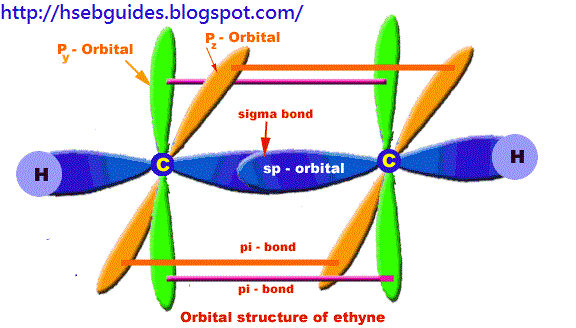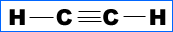# Ethyne

Chemistry of ethyne
Introcudtion
Molecular formula = C2H2
Empirical formula = CH
Molecular mass = 26
Empirical mass = 13
Common name = Acetylene
Homologous series = Alkynes

Orbital structure of ethyne
Compostion of ethyne molecule:
Ethyne molecule consists of two C-atoms and two H-atoms (C2H2).

Nature of hybridization:
In ethyne molecule, each carbon atom is Sp-hybridized. Due to Sp-hybridization, each carbon atom generates two Sp-hybrid orbitals. In this way there exists four Sp-orbital in ethyne. These Sp-orbital are arranged in linear geometry and 180o apart. Remaining py and pz unhybrid orbitals of each carbon atom lie perpendicular to the plane of Sp-orbitals.

Sigma bond formation:
One Sp-hybrid orbital of each carbon atom overlaps to produce one sigma bond between two C-atoms. The remaining one Sp-orbital of each C-atom overlaps with one H-atom to produce sigma bond.

Pi-bond formation:
Py and Pz orbital of two carbon atoms are un-hybrid and make parallel overlapping to produce pi-bond.

Bond length:
The C–H bond is 1.09A and C-C is 1.2A.o.

Bond angle:
HCC bond angle is 180o.Methods of preparation
By the hydroclysis of calcium carbide:
CaC2 + 2H2O == C2H2 + Ca(OH)2

By tetra chloroethane:
Cl2CH-CHCl2 + 2Zn == C2H2 + 2ZnCl2

By 1,2-dibromoethane:
Br-CH2-CH2-Br + KOH == C2H2 + 2KBr + 2H2O

Chemical properties
Combustion reaction:
2C2H2 + 5O2 == 4CO2 + 2H2O + heat

C2H2 == CH2=CH2 == C2H6

C2H2 +Cl2 == Cl-CH=CH-Cl == Cl2CH-CHCl2

C2H2 + HBr == CH2=CH-Br + HBr == CH3-CHBr2

C2H2 + HCN == CN-CH=CH-CN

C2H2 + HOH == CH2=CH-OH == [rearrangement] == CH3CHO (Ethanal)

Oxidation of ethyne:
In cold solution
C2H2 + HOH + 3[O] == 2HCOOH (Formic acid)
In hot solution,
C2H2 +4[O] == (COOH)2 (Oxalic acid)

Substitution reactions: